NEET  >  Test: Physics - 5

# Test: Physics - 5

Test Description

## 45 Questions MCQ Test NEET Mock Test Series | Test: Physics - 5

Test: Physics - 5 for NEET 2023 is part of NEET Mock Test Series preparation. The Test: Physics - 5 questions and answers have been prepared according to the NEET exam syllabus.The Test: Physics - 5 MCQs are made for NEET 2023 Exam. Find important definitions, questions, notes, meanings, examples, exercises, MCQs and online tests for Test: Physics - 5 below.
Solutions of Test: Physics - 5 questions in English are available as part of our NEET Mock Test Series for NEET & Test: Physics - 5 solutions in Hindi for NEET Mock Test Series course. Download more important topics, notes, lectures and mock test series for NEET Exam by signing up for free. Attempt Test: Physics - 5 | 45 questions in 45 minutes | Mock test for NEET preparation | Free important questions MCQ to study NEET Mock Test Series for NEET Exam | Download free PDF with solutions
 1 Crore+ students have signed up on EduRev. Have you?
Test: Physics - 5 - Question 1

### An electron moving with kinetic energy  6.6 x 10 -14J enters a magnetic field 4 x 10 -3 T at right angle to it. The radius of its circular path will be nearest to

Test: Physics - 5 - Question 2

### A closely wound flat circular coil of 25 turns of wire has diameter of 10 cm which carries current of 4 A, the flux density at the centre of a coil will be

Test: Physics - 5 - Question 3

### An electron is travelling along the x-direction. It encounters a magnetic field in the y-direction. Its subsequent motion will be

Test: Physics - 5 - Question 4
The magnetic Resonance Imaging (MRI) is based on the phenomenon of
Test: Physics - 5 - Question 5

A circular coil of radius R carries an electric current. The magnetic field due to the coil a point on the axis of the coil located at a distance r from the centre of the coil, such that r >> R, varies as

Detailed Solution for Test: Physics - 5 - Question 5

When r >> R Magnetic field at a point on the axis of a loop is given by B = (mu /2 * pi) (AI/ r³)Hence B is proportional to 1/r³ Hence Correct option is D.

Test: Physics - 5 - Question 6

A cyclotron is operating at a frequency of 12 x 106 Hz. Mass of deuteron is 3.3 x 10 -27 kg and charge on deuteron is  1.6 x 10 -19 induction of the necessary magnetic field is

Test: Physics - 5 - Question 7

The strength of the transverse magnetic field required to bend photoelectrons of maximum kinetic energy with in a circle of radius 50 cm when light of wavelength 4000Å is incident on barium emitter is (work function of barium is 2.5 eV)

Test: Physics - 5 - Question 8

Two equal electric currents are flowing in wires perpendicular to each other as shown in figure. AB and CD are lines perpendicular to each other and symmetrically placed with respect to currents. Then the resultant magnetic field will be zero on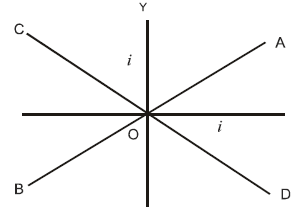Detailed Solution for Test: Physics - 5 - Question 8

Applying right hand grip rule and considering AB, the direction of magnetic field due to one current is upwards and that due to other is downwards. Both the magnetic fields cancel out each other, and the resultant magnetic field is zero. Considering CD and applying right hand grip rule for the two currents, the direction of magnetic field is in the same direction in both the cases giving non-zero resultant

Test: Physics - 5 - Question 9
A straight wire of diameter 0.5 mm carrying a current of 1 A is replaced by another wire of 1 mm diameter carrying the same current. The strength of magnetic field far away is
Test: Physics - 5 - Question 10

The semicircular portion (radius R) of a wire carrying current i is placed in a uniform magnetic field B. The magnetic field is perpendicular to the plane of the circle. The magnetic of the force on the wire is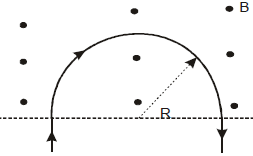Test: Physics - 5 - Question 11

A & B are two concentric circular conductors of centre O and carrying currents i1 & i2 as shown in the diagram. If ratio of their radii is 1:2 and ratio of the flux densities at O due to A and B is 1:3, then the value of i1 & i2 will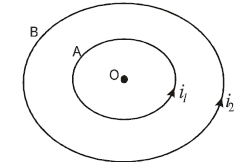Test: Physics - 5 - Question 12
Domain formation is the necessary feature of
Test: Physics - 5 - Question 13

A magnet 10 cm long and having a pole strength 2 A-m is deflected through 30º from the magnetic meridian. The horizontal component of earth’s induction is  0.32 x 10 -4T then the value of deflecting couple is

Test: Physics - 5 - Question 14

A spectrum of iron of permeability 8 x 10 -3 Wb / A-m  is placed in a magnetic field of strength 160 A/m. Then magnetic induction in this iron is

Test: Physics - 5 - Question 15

Two magnets each of magnetic moment M are placed so as to form a cross at right angles to each other. The magnetic moment of the system will be

Test: Physics - 5 - Question 16

At a certain place, the horizontal component of earth’s magnetic field is √3 times of its vertical component, the angle of dip at that place is

Test: Physics - 5 - Question 17

The work done in turning a magnet of magnetic moment M by an angle of 90ºfrom the meridian is n times the corresponding work done to turn it through an angle of 60º. The value of n is given by

Test: Physics - 5 - Question 18

The time of vibration of a dip needle vibrating in the vertical plane in the magnetic meridian is 3s.When the same magnetic needle is made to vibrate in the horizontal plane, the time is vibration is again 3s. Then angle of dip will be

Test: Physics - 5 - Question 19

A magnet makes 30 oscillations per minute in the earth’s magnetic field. If the magnetic field is doubled. The period of oscillation of magnet is

Test: Physics - 5 - Question 20

A moving conductor coil produces an induced emf. This is in accordance with

Test: Physics - 5 - Question 21

The varying current in a coil changes from 10 A to zero in 0.5 s. If the average emf induced in th coil is 220 V, then the self-inductance of the coil will be

Test: Physics - 5 - Question 22

Magnetic flux in a circuit containing a coil of resistance 2Ωchanges from 2.0 Wb to 10 Wb in 0.2 s. The charge passed through the coil in this time is

Test: Physics - 5 - Question 23
A metallic square loop ABCD is moving in its own plane with velocity v in a uniform magnetic field perpendicular to its plane as shown in the figure. An electric field is induced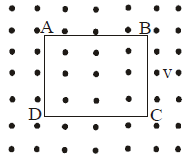Test: Physics - 5 - Question 24

In the circuit shown in the figure, what is the value of I1 just after pressing the key K?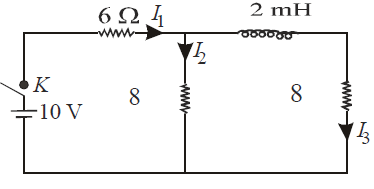Test: Physics - 5 - Question 25

A uniform but time-varying magnetic field B(t) exists in a cylindrical region of radius a and is directed into the plane of the paper, as shown. The magnitude of the induced electric field at point P at a distance r from the centre of the circular region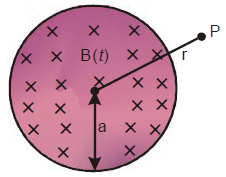Test: Physics - 5 - Question 26

A conducting rod AC of length 4I is rotated about a point O in a uniform mangetic field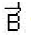directed into the paper. AO =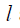and OC = 3. Then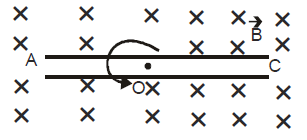Detailed Solution for Test: Physics - 5 - Question 26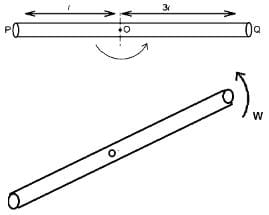Potential difference between point O & P is VO – VA.

Potential difference between point O & Q is VO – VC

Potential difference between point P & Q is

VA – VC = (VO – VC) – (VO – VA) (1)

Now for a rotating rod emf = BVℓ

Consider a small element of length dℓ angular velocity = ω = (V/ℓ) hence

dE = Bωℓ dℓ

∴ Total emf = O Bωℓ dℓ = [{Bωℓ2}/{2}]

∴ VO – VA = [{Bωℓ2}/{2}]

VO – VC = [{Bω(3ℓ)2}/ {2}]

From (1),
VA – VC = (9/2) Bωℓ2 – [{Bωℓ2} /{2}] = 4 Bωℓ2.

Test: Physics - 5 - Question 27

Switch S of the circuit shown in figure is closed at t = 0. If e denotes the induced emf in L and i , the current flowing through the circuit at time t, which of the following graphs is correct?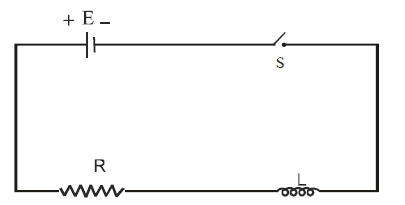Test: Physics - 5 - Question 28
A device for generating and alternating current of a desired frequency is known as
Test: Physics - 5 - Question 29

In an AC circuit containing only capacitance,the current

Test: Physics - 5 - Question 30

The rms value of emf of a saw totothed AC of peak voltage 60 volt is

Test: Physics - 5 - Question 31
In an ideal parallel L-C circuit, the capacitor is charged by connecting it to a DC source which is then disconnected. The current in the circuit
Test: Physics - 5 - Question 32

The rms value of AC voltage is  v 3+4 sin (100 πt )

Test: Physics - 5 - Question 33

Two coils C1 & C2 are placed adjacent to each other, if current is changed in coil C1 at a rate of 5A/sec, the emf induced in coil C2 is 20mV. What emf will be induced in 7C1 if the current is changed in Coil C2 at a rate of 7 A/Sec (Assume there is no change in orientation or position of the coils)

Test: Physics - 5 - Question 34

A magnetic needle is kept in a non uniform magnetic field. It experiences

Test: Physics - 5 - Question 35

A Cu ring is suspended by a thread in a vertical plane. The north pole of a magnet is brought near the ring in horizontal direction as shown in figure. What will be effect on the ring ?

Test: Physics - 5 - Question 36

An emf  E = 4 Cos 1000 t volt is applied to an L-R circuit of inductance 3 mH and resistance 4 Ω. The amplitude of the current in the circuit is

Test: Physics - 5 - Question 37

Direction : Read the following question and choose

A. If both Assertion and Reason are correct and reason is the correct explanation of assertion

B. If both Assertion and Reason are true, but Reason is not correct explanation of the Assertion

C. If Assertion is true, but the Reason is false

D. If Assertion is false, but the Reason is true

E. If both assertion and reason are false

Assertion : A.C. is more dangerous than D.C.

Reason: The peak value of A.C. is 41.4% higher than the D.C

Test: Physics - 5 - Question 38

Direction : Read the following question and choose

A. If both Assertion and Reason are correct and reason is the correct explanation of assertion

B. If both Assertion and Reason are true, but Reason is not correct explanation of the Assertion

C. If Assertion is true, but the Reason is false

D. If Assertion is false, but the Reason is true

E. If both assertion and reason are false

Assertion: Self Inductance is also known as inertia of electricity.

Reason : Self Inductance is the property of Coil due to which the coil opposes the flow current through it

Test: Physics - 5 - Question 39

Direction : Read the following question and choose

A. If both Assertion and Reason are correct and reason is the correct explanation of assertion

B. If both Assertion and Reason are true, but Reason is not correct explanation of the Assertion

C. If Assertion is true, but the Reason is false

D. If Assertion is false, but the Reason is true

E. If both assertion and reason are false
Assertion: In the phenomenon of mutual induction self induction of each of the coils persists
Reason: Self induction arises when strength of current in one coil changes. In this case, current may be changing in both the individual coils

Test: Physics - 5 - Question 40

Direction : Read the following question and choose

A. If both Assertion and Reason are correct and reason is the correct explanation of assertion

B. If both Assertion and Reason are true, but Reason is not correct explanation of the Assertion

C. If Assertion is true, but the Reason is false

D. If Assertion is false, but the Reason is true

E. If both assertion and reason are false
Assertion: When number of turns in a coil is doubled, coefficient of self-inductance of the coil becomes 4 times
Reason : This is because L ∞ N2

Test: Physics - 5 - Question 41

Direction : Read the following question and choose

A. If both Assertion and Reason are correct and reason is the correct explanation of assertion

B. If both Assertion and Reason are true, but Reason is not correct explanation of the Assertion

C. If Assertion is true, but the Reason is false

D. If Assertion is false, but the Reason is true

E. If both assertion and reason are false
Assertion: It is more difficult to push a magnet into a coil with more loops
Reason : This is because emf induced in each current loop resists the motion of the magnet

Test: Physics - 5 - Question 42

Direction : Read the following question and choose

A. If both Assertion and Reason are correct and reason is the correct explanation of assertion

B. If both Assertion and Reason are true, but Reason is not correct explanation of the Assertion

C. If Assertion is true, but the Reason is false

D. If Assertion is false, but the Reason is true

E. If both assertion and reason are false
Assertion :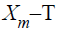graph for a diamagnetic material is a straight line parallel to T-axis

Reason : This is because susceptibility of a diamagnetic material is not affected by temperature

Test: Physics - 5 - Question 43

Direction : Read the following question and choose

A. If both Assertion and Reason are correct and reason is the correct explanation of assertion

B. If both Assertion and Reason are true, but Reason is not correct explanation of the Assertion

C. If Assertion is true, but the Reason is false

D. If Assertion is false, but the Reason is true

E. If both assertion and reason are false
Assertion : In an electric field, an electron beam perpendicular to field is deflected along a parabola, and in a magnetic field, the electron path perpendicular to the field will be circular
Reason: In electric field, force is perpendicular to path and along the field.In magnetic field, force is perpendicular to both, the magnetic field and path of beam

Test: Physics - 5 - Question 44

Direction : Read the following question and choose

A. If both Assertion and Reason are correct and reason is the correct explanation of assertion

B. If both Assertion and Reason are true, but Reason is not correct explanation of the Assertion

C. If Assertion is true, but the Reason is false

D. If Assertion is false, but the Reason is true

E. If both assertion and reason are false
Assertion: The energy of charged particle moving in a uniform magnetic field does not change
Reason: Work done by the magnetic field on the charge is zero

Test: Physics - 5 - Question 45

Direction : Read the following question and choose

A. If both Assertion and Reason are correct and reason is the correct explanation of assertion

B. If both Assertion and Reason are true, but Reason is not correct explanation of the Assertion

C. If Assertion is true, but the Reason is false

D. If Assertion is false, but the Reason is true

E. If both assertion and reason are false
Assertion: A linear solenoid carrying current is equivalent to a bar magnet
Reason: The magnetic field lines of both are same

## NEET Mock Test Series

1 videos|25 docs|144 tests
 Use Code STAYHOME200 and get INR 200 additional OFF Use Coupon Code
Information about Test: Physics - 5 Page
In this test you can find the Exam questions for Test: Physics - 5 solved & explained in the simplest way possible. Besides giving Questions and answers for Test: Physics - 5, EduRev gives you an ample number of Online tests for practice

## NEET Mock Test Series

1 videos|25 docs|144 tests

### How to Prepare for NEET

Read our guide to prepare for NEET which is created by Toppers & the best Teachers